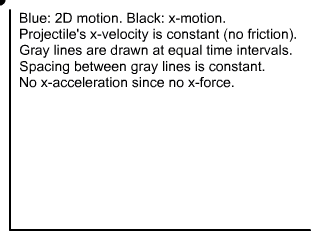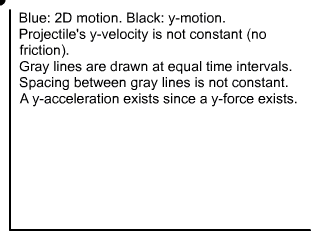﻿ Projectile Motion, X and Y Displacements | Zona Land Education

# Projectile Motion, X and Y Displacements

Time interval:

View markers:

This is an interactive animation that shows the x- and y-displacements for an object traveling in projectile motion without air resistance near the surface of a planet, such as Earth. You can adjust the following parameters:

• vxo - This is the original x-velocity of the projectile.
• vyo - This is the original y-velocity of the projectile.
• ay - This is the constant y-acceleration of the projectile, the acceleration due to gravity.
• Time interval - This is the length of time between which the displacement markers are drawn.
• View markers - These checkboxes control the visibility of their respective displacement markers.
• Pause/Resume - This button pauses or resumes the motion of the animation.

You should notice that the x-displacements are constant per time interval.This is because the x-velocity of the projectile is constant. The x-velocity is constant because there is no x-acceleration. There is no x-acceleration because there is no x-force. In other words, gravity does not pull sideways.

You should notice that the y-displacements change per second.This is because the y-velocity of the projectile is changing. The y-velocity is changing because there is a y-acceleration. There is a y-acceleration because there is a y-force. In other words, gravity pulls downward.